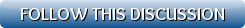[Show all top banners]
 Back to: Kurakani General Refresh page to view new repliesc - P R O G R A M I N G
 [VIEWED 2721 TIMES] SAVE! for ease of future access.
comingsoonPosted on 09-26-13 11:47 PM     Reply [Subscribe]
Login in to Rate this Post:     0?Write a function that receives an integer, b, that represents a number of bytes. If 1 ≤ b ≤ 8, the function will return the largest positive value that an unsigned int of b bytes can represent. If b is not in the appropriate range, the function should return -1. The function declaration is
long largest(int);
Assume that this function will be executed on a 64-bit operating system. You can use the pow() function which returns a double with the value b^e when called with
pow(b, e).

Can somebody help?

helpjava11Posted on 09-27-13 12:48 PM     [Snapshot: 157]     Reply [Subscribe]
Login in to Rate this Post:     0?Basically you need to understand the logic for this problem.
1 byte = 8 bit  ( Are sure you dont mean 8 bits, bit and byte are different)
You can find the largest possible integer value possible with any bit with this forlula: (2^n-1)
In your case  Total integer = 2^64 = 18446744073709551616
That means you can represent 0 to 18446744073709551615 with 8 bytes.

``#include<math.h> main() { double b = 64; b = pow(2,b); }``
This is not a complete code, try for yourself. If you still have doubt contact me